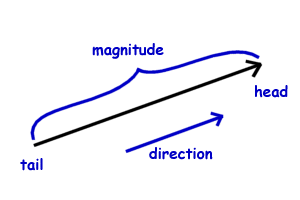Science >> Physics for Kids

# Physics for Kids

## Scalars and Vectors

There are a lot of different mathematical quantities used in physics. Examples of these include acceleration, velocity, speed, force, work, and power. These different quantities are often described as being either "scalar" or "vector" quantities. Below we will discuss what these words mean as well as introduce some basic vector math.

What is a scalar?

A scalar is a quantity that is fully described by a magnitude only. It is described by just a single number. Some examples of scalar quantities include speed, volume, mass, temperature, power, energy, and time.

What is a vector?

A vector is a quantity that has both a magnitude and a direction. Vector quantities are important in the study of motion. Some examples of vector quantities include force, velocity, acceleration, displacement, and momentum.

What is the difference between a scalar and vector?

A vector quantity has a direction and a magnitude, while a scalar has only a magnitude. You can tell if a quantity is a vector by whether or not it has a direction associated with it.

Example:

Speed is a scalar quantity, but velocity is a vector that specifies both a direction as well as a magnitude. The speed is the magnitude of the velocity. A car has a velocity of 40 mph east. It has a speed of 40 mph.How to Draw a Vector

A vector is drawn as an arrow with a head and a tail. The magnitude of the vector is often described by the length of the arrow. The arrow points in the direction of the vector. See the picture above.

How to Write a Vector

Vectors are generally written as boldface letters. They can also be written with an arrow over the top of the letter.

Example questions: Is it a scalar or a vector?

1) The football player was running 10 miles an hour towards the end zone.

This is a vector because it represents a magnitude (10 mph) and a direction (towards the end zone). This vector represents the velocity of the football player.

2) The volume of that box at the west side of the building is 14 cubic feet.

This is a scalar. It might be a bit tricky as it gives the location of the box at the west side of the building, but this has nothing to do with the direction of the volume which has a magnitude of 14 cubic feet.

3) The temperature of the room was 15 degrees Celsius.

This is a scalar, there is no direction.

4) The car accelerated north at a rate of 4 meters per second squared.

This is a vector as it has both direction and magnitude. We also know that acceleration is a vector quantity.

Interesting Facts about Scalars and Vectors
• Unit vectors are vectors with a magnitude of 1. They are used to define direction.
• The credit for inventing vectors is usually given to Irish physicist William Rowan Hamilton.
• Vectors and scalars are important in many fields of math and science.
• Vectors can be defined in two dimensional or three dimensional space.
• Vector graphics are sometimes used in computers because they can be scaled to a larger size without losing any image quality.
Activities

More Physics Subjects on Motion, Work, and Energy

Science >> Physics for Kids